# Fomin potential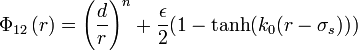$\Phi_{12}\left( r \right) = \left( \frac{d}{r} \right)^n + \frac{\epsilon}{2} (1 - \tanh ( k_0 (r-\sigma_s )))$
where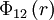$\Phi_{12}\left( r \right)$ is the intermolecular pair potential,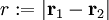$r := |\mathbf{r}_1 - \mathbf{r}_2|$ is the distance between site 1 and site 2,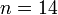$n=14$ and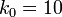$k_0=10$.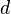$d$ is the diameter of the hard core,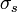$\sigma_s$ is the width of the repulsive shoulder,$\epsilon$ is the height of the shoulder. As such, this model can be viewed as a softened square shoulder model.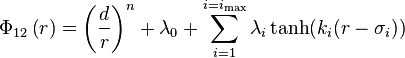$\Phi_{12}\left( r \right) = \left( \frac{d}{r} \right)^n + \lambda_0 + \sum_{i=1}^{i=i_{ \mathrm{max} }} \lambda_i \tanh ( k_i (r-\sigma_i ))$
By varying coefficients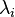$\lambda_i$ one can add repulsive shoulders or attractive wells to the potential.